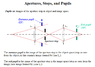# Calculating numerical aperture

• Aelo

## Homework Statement

An optical system consists of a thin lens in air with f = 100 mm. An aperture stop with a diameter of 20 mm is placed 25 mm to the right of the lens. Calculate the numerical aperture of the system. (Hint: Find the size of the entrance pupil.) What would be the numerical aperture if the aperture stop were placed 25 mm to the left of the lens?

## Homework Equations

NA = h_e/f
h_e is the height of the entrance pupil

## The Attempt at a Solution

I've drawn a picture, but I can't figure out how to find h_e. (The hint was rather useless given h_e appears in the relevant equation!) I know the entrance pupil is the image of the aperture stop in the object space (so you can actually see it?). Help is appreciated.

You know the distance to the aperture stop, and you know the size of it. If you use some simple geometry, you should be able to calculate the size of the entrance pupil, but this requires you understand, what it is?

I'm not sure I really do. I have a drawing from my book that shows the entrance pupil appearing somewhere between the lens and the aperture, and at some height above the aperture, but I have no idea how to determine either of these two values.

Well, from which side are you looking? To me it seems, like it doesn't matter, as you consider both in front and behind the lens. So unless it's given, I think you can see it, as if you are looking from whatever side you wish. So it should be obvious, if the entrance pupil is in front of the lens from the direction you are looking in.

Then when it is behind the lens, you probably need to bring some more formulas about the magnification from such a lens. It is in this case, I say you can use simple geometry to do this, but your book should even give you the formulas. What you need to look for, is some way to determine the position of the pupil, I think it looks like s'=s*f/(s-f) but I could be wrong. Then it should follow straight forward, how to calculate the magnification of the image, and then the numerical aperture.

Attached is the slide our professor gave us about it. I'm starting with trying it in front of the lens because that's the order in which the questions are asked. In the picture, the pupil image is in some rather ambiguous location between the lens and the aperture and above the aperture, and I can't find any equations telling me how to relate the distance between the lens and the aperture and the aperture height to where the pupil ends up. The equation you mentioned is for the distance from the lens to the image (with s being the distance from the lens to the object).

#### Attachments

•Untitled.png
13.1 KB · Views: 633
Ahh, sorry, now I think I understand it correctly :P

So you have a lens, and you have an aperture stop. When you look through this system, the aperture stop will limit, whatever image you would be looking at. And this "image" is defined as the entrance pupil. You could even say, it's an image of the aperture stop.
I recommend looking at hyperphysics: http://hyperphysics.phy-astr.gsu.edu/hbase/geoopt/stop.html
Anyway, this means you can use the formula I mentioned last, since you know the distance, s, to the aperture stop. And then you can go on with the recipe, I mentioned.

•1 person
The link you posted is very helpful, and I saw that the entrance pupil was formed by the continuation of the extreme ray, but since that continuation doesn't actually exist (as the ray ended up being redirected by the lens), how is the image visible? What rays make it exist?

I see how you can use the s' formula, since it is in fact measuring the distance from the lens to the image (the image being the entrance pupil, in this case). The equation I have in my notes has a plus sign in the denominator... giving s' = (25mm*100mm)/(25mm + 100mm) = 20mm
M = s'/s = 20mm/25mm = 0.8

How do we relate NA to M? I'm thinking that the image is 0.8 times the height of the object, so h_e is 0.8*20mm = 16mm?

Yes that is how, I would do it.
But I do find your formula a bit strange, isn't it usually a negative sign in the denominator (f*s/(f-s))=s'
surely I could be wrong, but the formula usually depends on the type of lens. So you should make sure, it is the correct type of lens, you use the formula for. Though if no more information is given, that could be difficult.

•1 person
I think it has to do with sign convention... the formula is usually written 1/f = 1/l' + 1/l, but in my class, it's written 1/f = 1/l' - 1/l.

For where it says, what if aperture stop were placed 25 mm to the left, is that just changing the sign of l and that's all?

(Also, you've been very helpful and I appreciate it a great deal. I asked another question from this class a day ago, and I know no one has to help me, but if you have the time, please take a look? Your explanations are very clear. https://www.physicsforums.com/showthread.php?p=4510894)

Last edited:
well that depends on your coordinate system. The formula you mention is correct with the positive sign, and if you isolate it for l', you get the formula (l*f)/(f-l)=l'

Thank you very much for your help, I believe I have solved the problem. :)